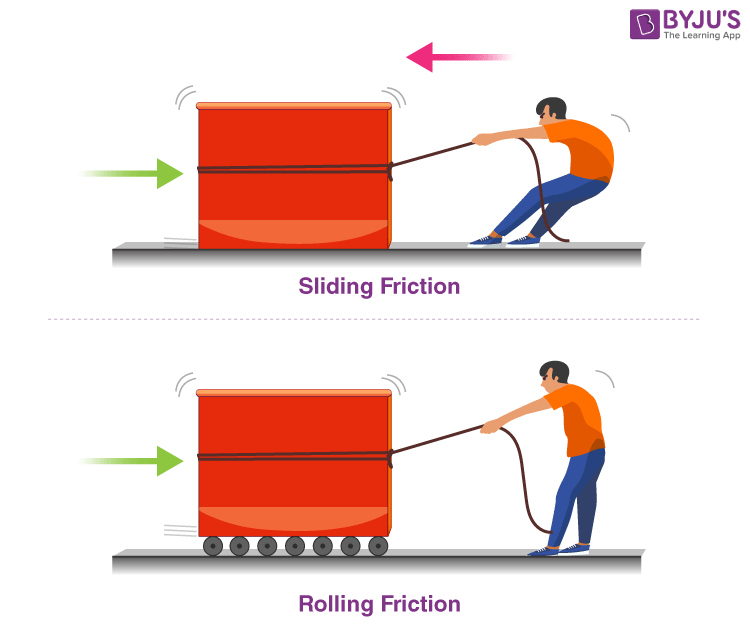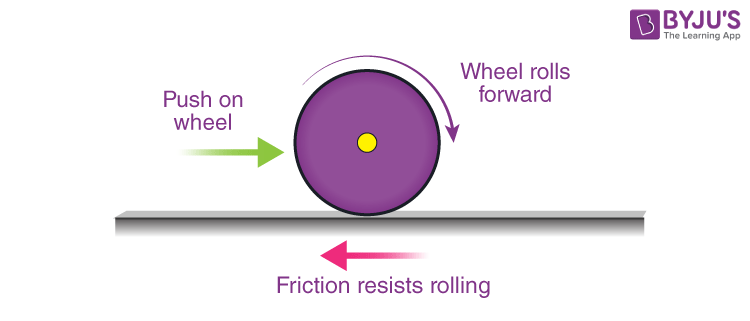# Rolling Friction

Rolling friction is a type of force that opposes the motion of a rolling body. In this article, let us learn in detail about rolling friction and the coefficient of rolling friction.

## What Is Rolling Friction?

For a moving solid body, there are two principal types of friction that act upon it:

• The force resisting the motion of a rolling body on a surface is known as rolling friction or rolling resistance. Rolling of ball or wheel on the ground is an example of Rolling friction.
• The other type of friction is sliding friction. In this type of friction, there is a restriction on the body’s movement as only one side of the body is in contact with the surface. Pushing a box across the table is an example of Sliding friction.

Rolling friction is considerably weaker than sliding friction.### Laws of Rolling Friction

There are three laws of rolling friction:

• With the increase in smoothness, the force of rolling friction decreases.
• Rolling friction is expressed as a product of load and constant to the fractional power.
 F = kLn
• Rolling friction force is directly proportional to load and inversely proportional to the radius of curvature.
 $$\begin{array}{l}F=\mu \times \frac{W}{r}\end{array}$$

## Cause of Rolling Friction

When an object is rolled on a surface, certain things happen:

1. The object is deformed at the point of contact with the surface.
2. The surface is deformed at the point of contact with the object.
3. The motion is created below the surface as a result of the above-mentioned points.The primary cause of this friction is that the energy of deformation is greater than the energy of recovery. Also, there is an adhesive force between the two surfaces which needs to be overcome constantly. The amount of friction is based on a variety of factors such as:

• The quality of the sliding body
• The quality of the surface
• The diameter of the rolling object
• The surface area of the body

## Coefficient of Rolling Friction

Determining the coefficient of this friction is considerably more complex than that of sliding friction.

“Coefficient of Rolling Friction is the ratio of the force of rolling friction to the total weight of the object.”

In empirical terms, the coefficient of rolling resistance can be expressed as:

 $$\begin{array}{l}F_{r} = μ_{r}W\end{array}$$

where,

• Fr is the resistive force of rolling resistance
• μr is the coefficient of rolling resistance
• W is the weight of the rolling body

## Rolling Friction Examples

• A basketball rolled on the court will eventually come to a halt because of rolling friction.
• A bike with a broad tire will burn more fuel because of the increased rolling friction.
• A ball rolled on a field will go lesser distance than a ball rolling on a concrete floor because it will experience greater rolling friction on the former surface.

### What Is the difference between Rolling Friction and Sliding Friction?

Following is the table with rolling friction vs sliding friction:

 Rolling friction Sliding friction Rolling friction takes place when an object rolls on the surface. Sliding friction takes place when two surfaces are rubbed against each other. Rolling friction takes place due to the deformation of surfaces. Sliding friction takes place due to interlocking between microscopic surfaces. The coefficient of rolling friction is dependent on the radius of the rolling object, the depth to which the object can sink, and the toughness of the surface. The coefficient of sliding friction depends on the texture of the surface and temperature to a certain extent. It is independent of external factors. Coefficient of rolling friction: Fr = μrN Coefficient of sliding friction: Fk = μrN

## Frequently Asked Questions – FAQs

Q1

### What is friction?

Friction is the force that opposes the rolling or sliding of one solid body over another.
Q2

### What is meant by kinetic friction?

Kinetic friction is the friction that acts between moving surfaces.
Q3

### What is meant by static friction?

Static friction is the friction that acts between two stationary surfaces.
Q4

### What is meant by rolling friction?

Rolling friction is the force opposing the movement of a rolling object on a surface.
Q5

### Give an example of rolling friction.

A football rolling on the ground will slow down as time passes due to rolling friction.